• +91 9971497814
• info@interviewmaterial.com

# RD Chapter 16- Surface Areas and Volumes Ex-16.2 Interview Questions Answers

### Related Subjects

Question 1 : A tent is in the form of a right circular cylinder surmounted by a cone. The diameter of cylinder is 24 m. The height of the cylindrical portion is 11 m while the vertex of the cone is 16 m above the ground. Find the area of canvas required for the tent.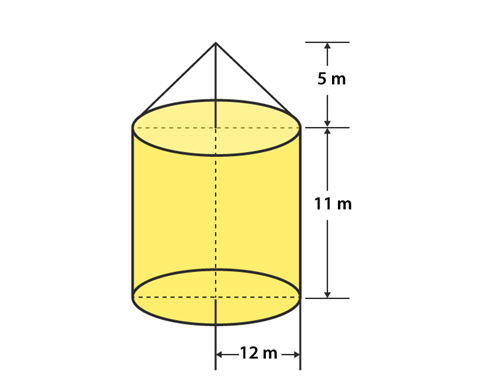Given,

The diameter of thecylinder (also the same for cone) = 24 m.

So, its radius (R)= 24/2 = 12 m

The height of theCylindrical part (H1) = 11m

So, Height of the conepart (H2) = 16 – 11 = 5 m

Now,

Vertex of the coneabove the ground = 11 + 5 = 16 m

Curved Surface area ofthe Cone (S1) = πRL = 22/7 × 12 × L

The slant height (L)is given by,

L = √(R2 +H22) = √(122 + 52) = √169

L = 13 m

So,

Curved Surface Area ofCone (S1) = 22/7 × 12 × 13

And,

Curved Surface Area ofCylinder (S2) = 2πRH1

S=2π(12)(11) m2

Thus, the area ofCanvas required for tent

S = S1 +S= (22/7 × 12 × 13) + (2 × 22/7 × 12 × 11)

S = 490 + 829.38

S = 1319.8 m2

S = 1320 m2

Therefore, the area ofcanvas required for the tent is 1320 m2

Question 2 : A rocket is in the form of a circular cylinder closed at the lower end with a cone of the same radius attached to the top. The cylinder is of radius 2.5 m and height 21 m and the cone has the slant height 8 m. Calculate the total surface area and the volume of the rocket.

Given,

Radius of thecylindrical portion of the rocket (R) = 2.5 m

Height of thecylindrical portion of the rocket (H) = 21 m

Slant Height of theConical surface of the rocket (L) = 8 m

Curved Surface Area ofthe Cone (S1) = πRL = π(2.5)(8)= 20π

And,

Curved Surface Area ofthe Cone (S2) = 2πRH + πR2

S=(2π × 2.5 × 21) + π (2.5)2

S2 =(π × 105) + (π × 6.25)

Thus, the total curvedsurface area S is

S = S1 +S2

S = (π20) + (π105) +(π6.25)

S = (22/7)(20 + 105 +6.25) = 22/7 x 131.25

S = 412.5 m2

Therefore, the totalSurface Area of the Conical Surface = 412.5 m2

Now, calculating thevolume of the rocket

Volume of the conicalpart of the rocket (V1) = 1/3 × 22/7 × R2 × h

V1 = 1/3× 22/7 × (2.5)2 × h

Let, h be the heightof the conical portion in the rocket.

We know that,

L2 = R+h2

h2 = L–R2 = 82 – 2.52

h = 7.6 m

Using the value of h,we will get

Volume of the conicalpart (V1) = 1/3 × 22/7 × 2.52 × 7.6 m2   =49.67 m2

Next,

Volume of theCylindrical Portion (V2) = πR2h

V2 = 22/7× 2.52 × 21 = 412.5 m2

Thus, the total volumeof the rocket = V1 + V2

V = 412.5 + 49.67 =462.17 m2

Hence, the totalvolume of the Rocket is 462.17 m

Question 3 : A tent of height 77 dm is in the form of a right circular cylinder of diameter 36 m and height 44 dm surmounted by a right circular cone. Find the cost of the canvas at Rs. 3.50 per m2

Given,

Height of the tent =77 dm

Height of a surmountedcone = 44 dm

Height of theCylindrical Portion = Height of the tent – Height of the surmounted Cone

= 77 – 44

= 33 dm = 3.3 m

And, given diameter ofthe cylinder (d) = 36 m

So, its radius (r) ofthe cylinder = 36/2 = 18 m

Let’s consider L asthe slant height of the cone.

Then, we know that

L2 = r2 +h2

L2 =182 + 3.32

L=324 + 10.89

L2 = 334.89

L = 18.3 m

Thus, slant height ofthe cone (L) = 18.3 m

Now, the CurvedSurface area of the Cylinder (S1) = 2πrh

S1 =2π (184.4) m

And, the CurvedSurface area of the cone (S2) = πrL

S= π× 18 × 18.3 m

So, the total curvedsurface of the tent (S) = S+ S2

S = S1 +S2

S = (2π18 × 4.4)+ (π18 × 18.3)

S = 1533.08 m2

Hence, the totalCurved Surface Area (S) = 1533.08 m2

Next,

The cost of 1 m2 canvas= Rs 3.50

So, 1533.08 m2 ofcanvas will cost = Rs (3.50 x 1533.08)

= Rs 5365.8

Question 4 : A toy is in the form of a cone surmounted on a hemisphere. The diameter of the base and the height of the cone are 6 cm and 4 cm, respectively. Determine the surface area of the toy.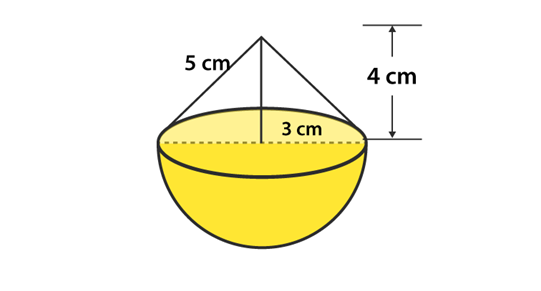Given that,

The height of the cone(h) = 4 cm

Diameter of the cone(d) = 6 cm

So, its radius (r) = 3

Let, ‘l’ be the slantheight of cone.

Then, we know that

l2 = r2 +h2

l2 = 32 +42 = 9 + 16 = 25

l = 5 cm

Hence, the slantheight of the cone (l) = 5 cm

So, the curved surfacearea of the cone (S1) = πrl

S1 = π(3)(5)

S1 =47.1 cm2

And, the curvedsurface area of the hemisphere (S2) = 2πr2

S2 = 2π(3)2

S2 =56.23 cm2

So, the total surfacearea (S) = S+ S2

S = 47.1 + 56.23

S = 103.62 cm2

Therefore, the curvedsurface area of the toy is 103.62 cm2

Question 5 : A solid is in the form of a right circular cylinder, with a hemisphere at one end and a cone at the other end. The radius of the common base is 3.5 cm and the height of the cylindrical and conical portions are 10 cm and 6 cm, respectively. Find the total surface area of the solid. (Use π = 22/7).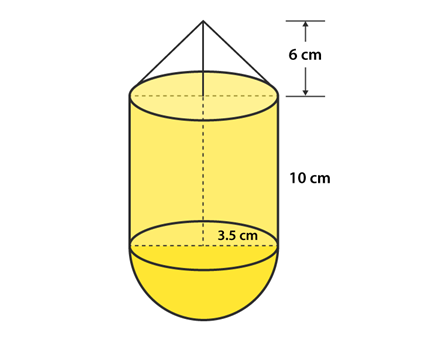Given,

Radius of the commonbase (r) = 3.5 cm

Height of thecylindrical part (h) = 10 cm

Height of the conicalpart (H) = 6 cm

Let, ‘l’ be the slantheight of the cone

Then, we know that

l2 = r2 +H2

l2 =3.52 + 62 = 12.25 + 36 = 48.25

l = 6.95 cm

So, the curved surfacearea of the cone (S1) = πrl

S1 = π(3.5)(6.95)

S1 =76.38 cm

And, the curvedsurface area of the hemisphere (S2) = 2πr2

S2 = 2π(3.5)2

S2 =77 cm2

Next, the curvedsurface area of the cylinder (S3) = 2πrh

S2 = 2π(3.5)(10)

S2 =220 cm2

Thus, the totalsurface area (S) = S+ S2 + S3

S = 76. 38 + 77 + 220= 373.38 cm2

Therefore, the totalsurface area of the solid is 373.38 cm2

Question 6 : A toy is in the shape of a right circular cylinder with a hemisphere on one end and a cone on the other. The radius and height of the cylindrical parts are 5cm and 13 cm, respectively. The radii of the hemispherical and conical parts are the same as that of the cylindrical part. Find the surface area of the toy if the total height of the toy is 30 cm.

Given,

Height of theCylindrical portion (H) = 13 cm

Radius of theCylindrical portion (r) = 5 cm

Height of the wholesolid = 30 cm

Then,

The curved surfacearea of the cylinder (S1) = 2πrh

S=2π(5)(13)

S=408.2 cm2

Let, ‘L’ be the slantheight of the cone

And, the curvedsurface area of the cone (S2) = πrL

S2 =π(6)L

For conical part, wehave

h = 30 – 13 – 5 = 12cm

Then, we know that

L2 = r2 +h2

L2 = 52 +122

L=25 + 144

L2 =169

L = 13 m

So,

S2 =π(5)(13) cm2

S2 =204.28 cm2

Now, the curvedsurface area of the hemisphere (S3) = 2πr2

S3 =2π(5)2

S3 =157.14 cm2

Thus, the total curvedsurface area (S) = S+ S+ S3

S = (408.2 + 204.28 +157.14)

S = 769.62 cm2

Therefore, the surfacearea of the toy is 770 cm2

Question 7 : Consider a cylindrical tub having radius as 5 cm and its length 9.8 cm. It is full of water. A solid in the form of a right circular cone mounted on a hemisphere is immersed in tub. If the radius of the hemisphere is 3.5 cm and the height of the cone outside the hemisphere is 5 cm, find the volume of water left in the tub.

Given,

The radius of theCylindrical tub (r) = 5 cm

Height of theCylindrical tub (H) = 9.8 cm

Height of the coneoutside the hemisphere (h) = 5 cm

Radius of the hemisphere= 3.5 cm

Now, we know that

The volume of theCylindrical tub (V1) = πr2H

V1 =π(5)9.8

V1 =770 cm3

And, the volume of theHemisphere (V2) = 2/3 × π × r3

V= 2/3× 22/7 × 3.53

V2 =89.79 cm3

And, the volume of theHemisphere (V3) = 23 × π × r × 2h

V= 2/3× 22/7 × 3.52 × 5

V=64.14 cm3

Thus, total volume (V)= Volume of the cone + Volume of the hemisphere

= V+V3

V = 89.79 + 64.14 cm

= 154 cm3

So, the total volumeof the solid = 154 cm3

In order to find thevolume of the water left in the tube, we have to subtract the volume of thehemisphere and the cone from the volume of the cylinder.

Hence, the volume ofwater left in the tube = V– V2

= 770 – 154

= 616 cm3

Therefore, the volumeof water left in the tube is 616 cm3.

Question 8 : A circus tent has a cylindrical shape surmounted by a conical roof. The radius of the cylindrical base is 20 cm. The heights of the cylindrical and conical portions is 4.2 cm and 2.1 cm respectively.  Find the volume of that tent.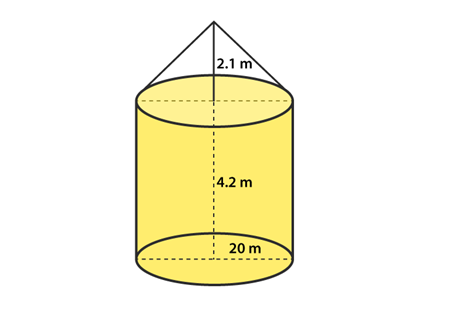Given,

Radius of thecylindrical portion (R) = 20 m

Height of thecylindrical portion (h1) = 4.2 m

Height of the conicalportion (h2) = 2.1 m

Now, we know that

Volume of theCylindrical portion (V1) = πrh1

V1 =π(20)24.2

V=5280 m3

And, the volume of theconical part (V2) = 1/3 × 22/7 × r2 × h2

V= 13× 22/7 × 202 × 2.1

V=880 m3

Thus, the total volumeof the tent (V) = volume of the conical portion + volume of the Cylindricalportion

V = V+V2

V = 6160 m3

Therefore, volume ofthe tent is 6160 m3

Question 9 : A petrol tank is a cylinder of base diameter 21 cm and length 18 cm fitted with the conical ends each of axis length 9 cm. Determine the capacity of the tank.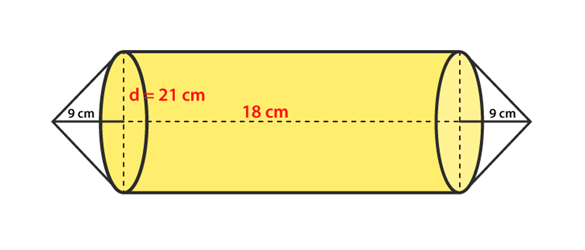Given,

Base diameter of thecylindrical base of the petrol tank = 21 cm

So, its radius (r)= diameter/2 = 21/2 = 10.5 cm

Height of theCylindrical portion of the tank (h1) = 18 cm

Height of the Conicalportion of the tank (h2) = 9 cm

Now, we know that

The volume of theCylindrical portion (V1) = πrh1

V1 =π(10.5)18

V1 =6237 cm3

The volume of theConical portion (V2) = 1/3 × 22/7 × r2 × h2

V= 1/3× 22/7 × 10.52 × 9

V=1039.5 cm3

Therefore, the totalvolume of the tank (V) = 2 x volume of a conical portion + volume of theCylindrical portion

V = V+V2 = 2 x 1039.5 + 6237

V = 8316 cm3

So, the capacity ofthe tank = V = 8316 cm

Question 10 : A conical hole is drilled in a circular cylinder of height 12 cm and base radius 5 cm. The height and base radius of the cone are also the same. Find the whole surface and volume of the remaining Cylinder.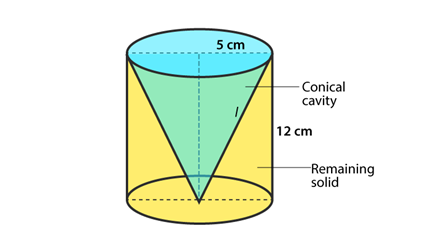Given,

Height of the circularCylinder (h1) = 12 cm

Base radius of thecircular Cylinder (r) = 5 cm

Height of the conicalhole = Height of the circular cylinder, i.e., h= h=12 cm

And, Base radius ofthe conical hole = Base radius of the circular Cylinder = 5 cm

Let’s consider, L asthe slant height of the conical hole.

Then, we know that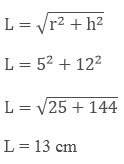Now,

The total surface areaof the remaining portion in the circular cylinder (V1) = πr+2πrh + πrl

V=π(5) + 2π(5)(12) + π(5)(13)

V=210 π cm2

And, the volume of theremaining portion of the circular cylinder = Volume of the cylinder – Volume ofthe conical hole

V = πr2h– 1/3 × 22/7 × r2 × h

V = π(5)2(12)– 1/3 × 22/7 × 52 × 12

V = 200 π cm2

Todays Deals### RD Chapter 16- Surface Areas and Volumes Ex-16.2 Contributorskrishan

Name:
Email:

# Latest News# 9000 interview questions in different categories